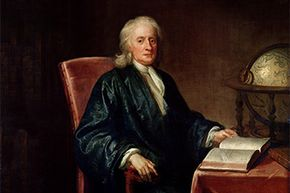# How Newton's Laws of Motion Work

By: William Harris  |Sir Isaac Newton was an intellectual rock star (as well as a snazzy dresser).

Next to E = mc², F = ma is the most famous equation in all of physics. Yet many people remain mystified by this fairly simple algebraic expression. It's actually a mathematical representation of Isaac Newton's second law of motion, one of the great scientist's most important contributions. The "second" implies that other laws exist, and, luckily for students and trivia hounds everywhere, there are only two additional laws of motion. All three are presented here, using Newton's own words:

1. Every object persists in its state of rest or uniform motion -­ in a straight line unless it is compelled to change that state by forces impressed on it.
2. Force is equal to the change in momentum per change in time. For a constant mass, force equals mass times acceleration.
3. For every action, there is an equal and opposite reaction.

These three laws form the foundation of what is known as classical mechanics, or the science concerned with the motion of bodies being acted upon by forces. The bodies in motion could be large objects, such as orbiting moons or planets, or they could be ordinary objects on Earth's surface, such as moving vehicles or speeding bullets. Even bodies at rest are fair game.